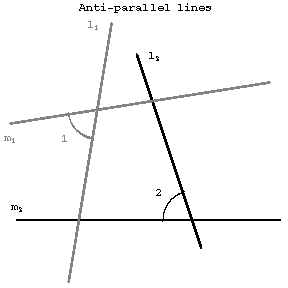# Anti-parallel straight lines

Template:OldImage with respect to two given lines \$m_1\$ and \$m_2\$

Two straight lines \$l_1\$ and \$l_2\$ which intersect \$m_1\$ and \$m_2\$ so that \$\angle1=\angle2\$ (cf. Fig.).Figure: a012660a

If \$l_1\$ and \$l_2\$ are anti-parallel with respect to \$m_1\$ and \$m_2\$, then \$m_1\$ and \$m_2\$ are also anti-parallel with respect to \$l_1\$ and \$l_2\$. In any quadrilateral inscribed in a circle, any two opposite sides are anti-parallel with respect to the other two sides. If the lines \$m_1\$ and \$m_2\$ intersect at a point \$O\$, one also says that \$l_1\$ and \$l_2\$ are anti-parallel with respect to the angle \$m_1Om_2\$. If the lines \$m_1\$ and \$m_2\$ coincide, \$l_1\$ and \$l_2\$ are said to be anti-parallel with respect to a straight line.

How to Cite This Entry:
Anti-parallel straight lines. Encyclopedia of Mathematics. URL: http://encyclopediaofmath.org/index.php?title=Anti-parallel_straight_lines&oldid=53264
This article was adapted from an original article by A.B. Ivanov (originator), which appeared in Encyclopedia of Mathematics - ISBN 1402006098. See original article